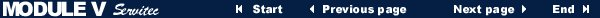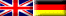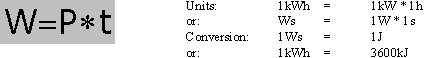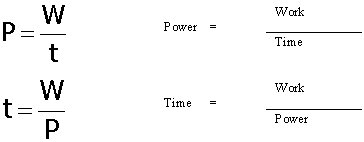MODULE V Electrical engineering - Basics Page 183. Electrical work, power, efficiency

3.1 The electrical work

 Measurement unit Ws Watt-second (James Watt, English Engineer, 1736-1819) Measurement unit J Joule (James Prescott Joule, English Physicist, 1818-1889) Unit symbol W Work

If an electric circuit is closed electrons are flowing. The resulting electrical energy can be used. If e.g. a water pump motor forms part of the closed electric circuit as a resistor, then electric energy is converted into mechanical energy. The motor is pumping heating water and doing so it performs a mechanical work.
The pump has to be supplied with electric energy in order to work. Such a use of electric energy is also called "electricity consumption" (however, more appropriately would be "electricity use").
The mechanical work that results from the electricity use is the product of electric power and consumption time. So, the electric work depends on the consumed electric power and on the time of the power consumption. That means that the bigger the electric work is the bigger has to be the power consumption and the longer the power consumption has to take place:

The following formula applies: Work = Power * TimeThe formula also can be transformed as follows:The quantity of the used electric work can be determined with electricity meters. Normally, the electricity consumer then pays a corresponding fee to the power supply company for his electricity consumption.• • Copyright © IWB e.V. 1996-2000 • Design, technical realisation and webmaster: ohlendorf@ohlendorf.de • •
• • Sponsored by EDUVINET • 800 x 600 recommended • •